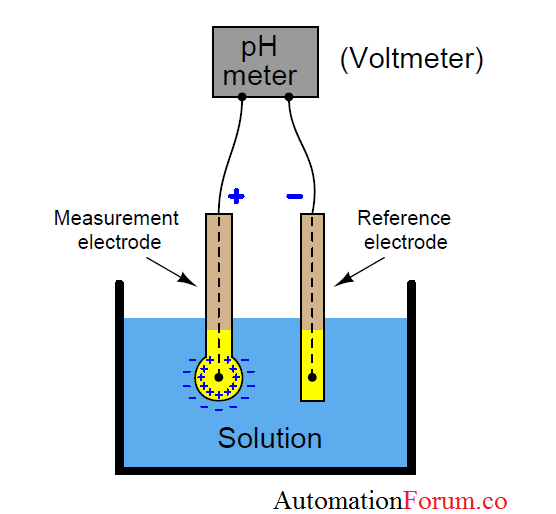# pH measurement : Glass electrode methodpH is the measure of the hydrogen ion concentration in a solution. It describes the degree of acidity or alkalinity (basicity) of a solution. pH measurement is used most often industrially with, but not limited to, aqueous (water-based) applications. The units of measure scale in most common applications ranges from 0 to 14 pH. 0 pH represents 1 (100) mole/liter of hydrogen, while 14 pH represents 0.0000000000001 (10-14) moles/liter of hydrogen. There is a factor of ten difference between each pH unit.

## Principle:

The pH value of a solution is measured using a pH electrode. It consists essentially of a pair of electrodes, measurement and reference electrode, both submerged in the solution of unknown pH. These two electrodes essentially form two half cells. Although the potential developed in the reference cell is constant, the potential of the measurement cell depends on the concentration of hydrogen ions in the solution and is governed by the Nernst equation:E= e.m.f of the half-cell
E0= e.m.f of the half-cell under saturated condition
R= Gas constant (8.314 J/ 0 C)
T= Absolute temperature (K)
N= valance of the ion
F= Faraday Constant = 96493 C
a= Activity co-efficient (0 1) ≤ ≤ a ; for a very dilute solution, a →1
C= molar concentration of ions.

## Working and Construction:

There are various methods of measuring pH, but the main methods are those using chemical indicators, hydrogen electrodes, antimony electrodes, or glass electrode. Here glass electrode method is described.

pH measurements can be most accurately made with a pH measurement “loop” consisting of a probe, a preamplifier, and an analyzer. The probe will have two or three modules – a measuring electrode, reference electrode, and a temperature compensator – which are required to make the measurement, as shown in below picture:This method employs the electromotive force that is generated on both sides of a glass membrane when the membrane separates two different solutions. This electromotive force is proportional to the difference in the pH of
the two solutions.The measuring electrode, which is sensitive to hydrogen ions, develops a potential (voltage) directly related to the hydrogen ion concentration in the solution. The reference electrode contains a fill solution, which slowly flows out of the electrode through the reference junction. This fill solution makes contact with the solution and the measuring electrode, completing the circuit.

Thus the voltage between the reference electrode, which should remain at a constant voltage, and the measuring electrode will change proportionally to the hydrogen ion concentration.

### Applications of pH measurement:

• For the diagnosis of various disorders in the human body
• Pharmaceutical
• Agriculture
• Corrosion prevention
• Dyeing
• Printing
Scroll to Top